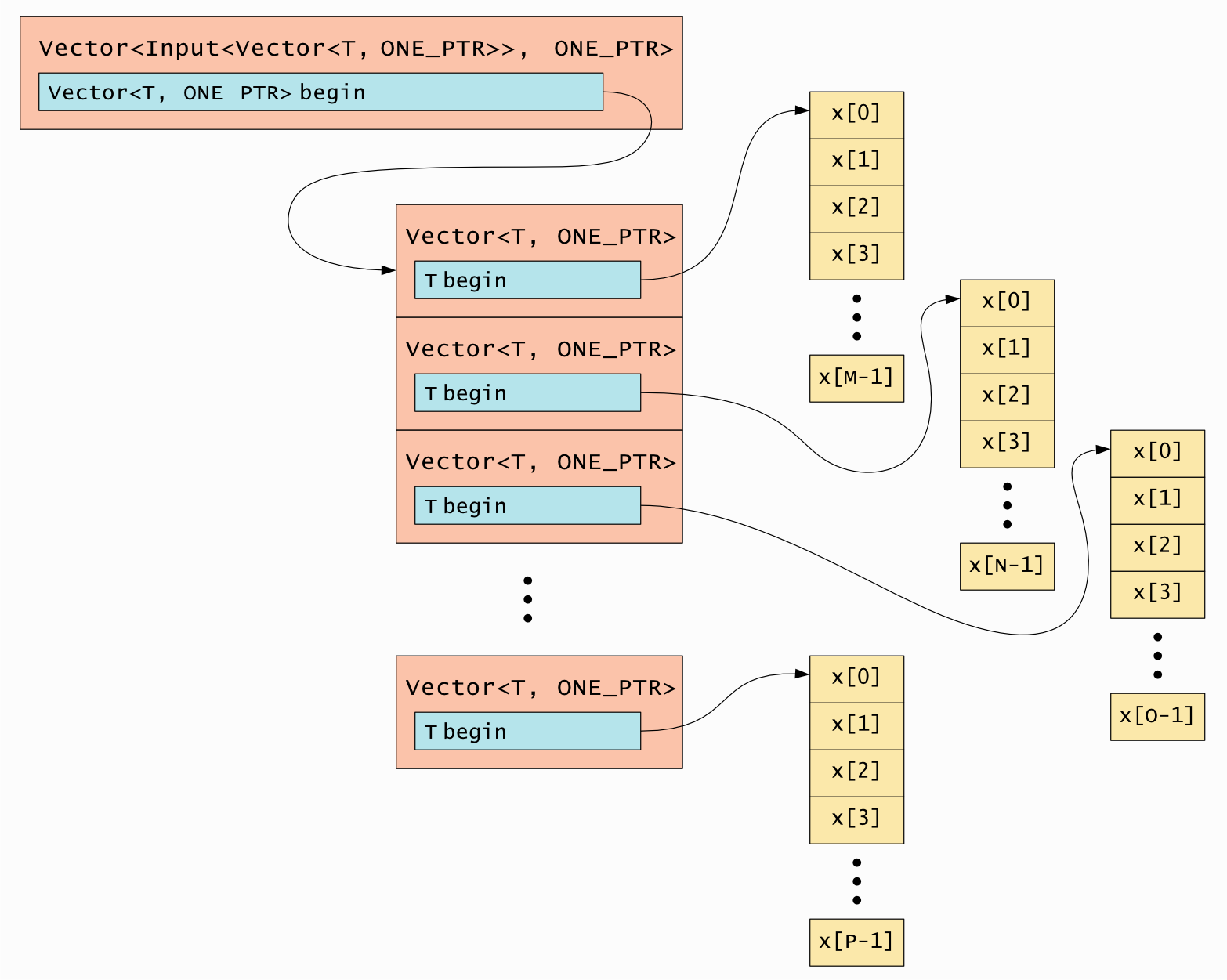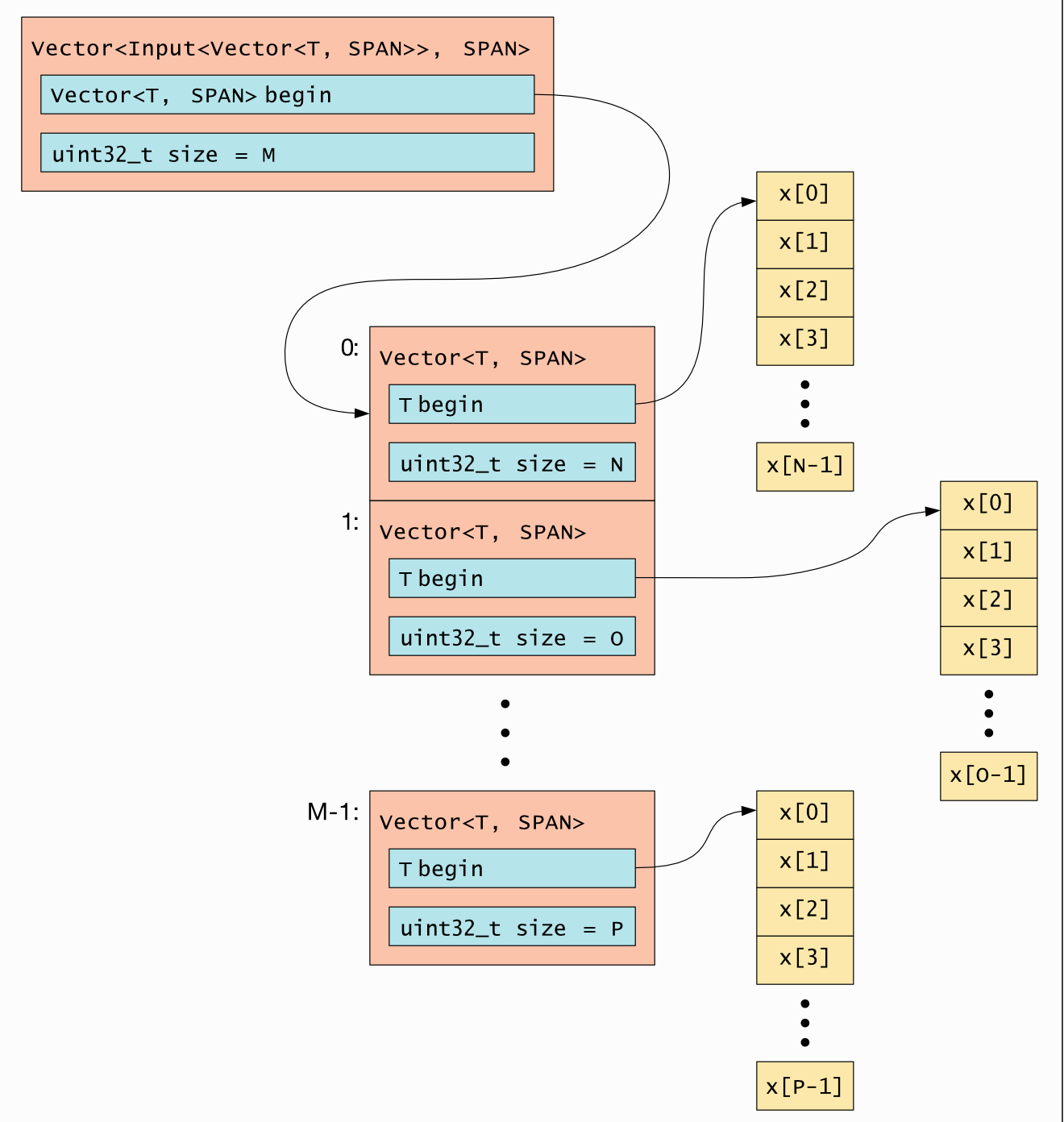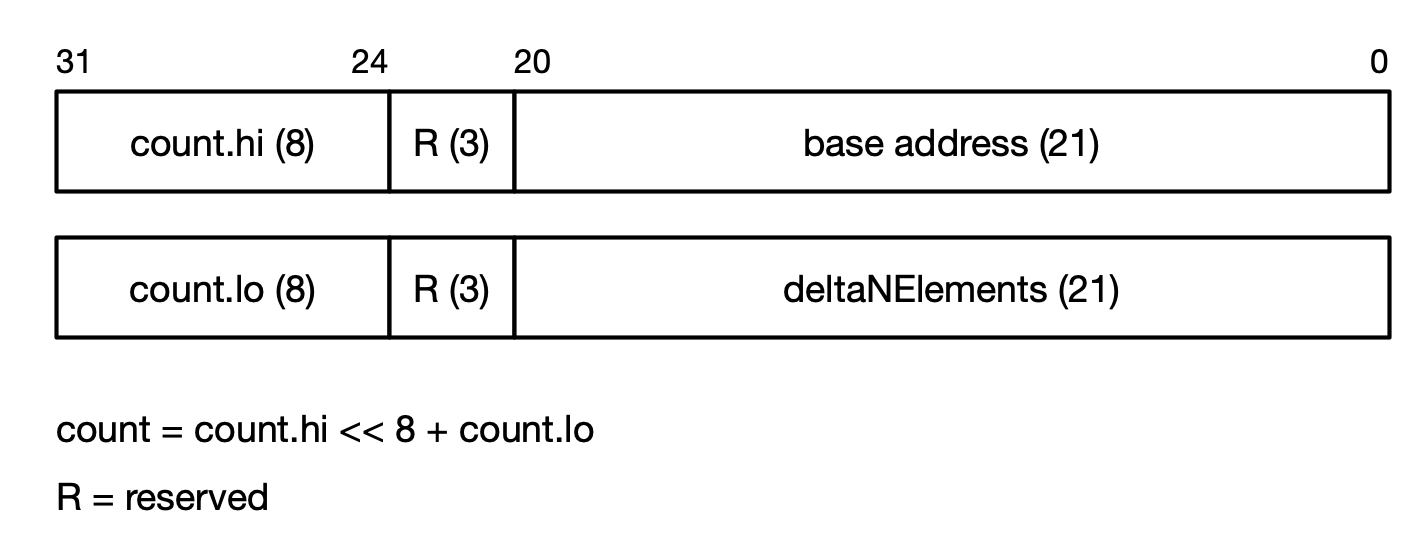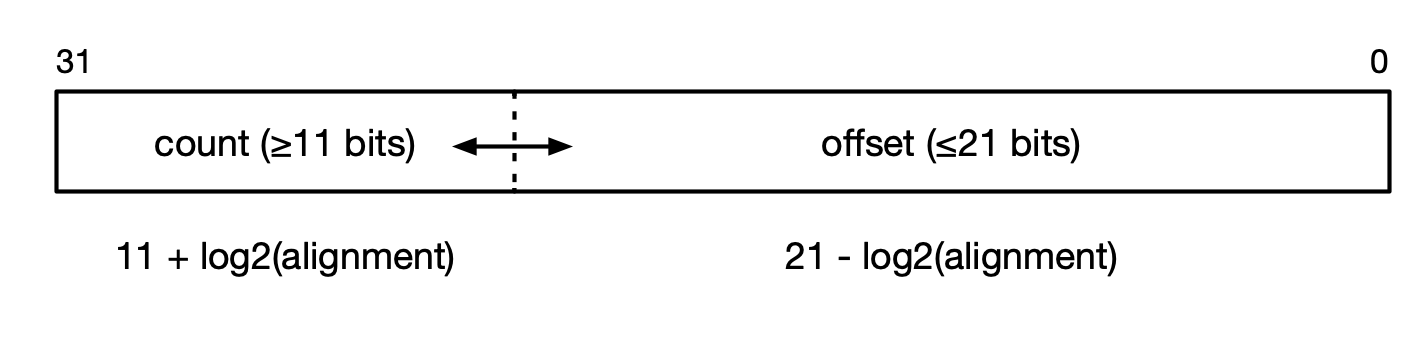# 4. Vector types¶

The fields of a Vertex can include Vector<T> or VectorList<T> types, or a combination of those such as Vector<Input<Vector<T>>>, in its state fields. These are similar to std::vector but can have different layouts in memory, optimised for the tile architecture.

These types are documented in the runtime API section of the Poplar and PopLibs API Reference.

## 4.1. Parameters¶

As well as the data type, the Vector and VectorList templates also have parameters to specify minimum alignment of elements, and whether or not they need to be stored in interleaved memory, for example:

template <typename T, VectorLayout L, unsigned MinAlign, bool Interleaved>
class Input<Vector<T, L, MinAlign, Interleaved>>
...


### 4.1.1. Types¶

The vector data type (T) can be any of the supported Poplar types defined in Types.hpp.

### 4.1.2. Layout¶

The template parameter L defines the type of memory layout to use. The valid layouts for a Vector are shown in Table 4.1. Some of these layouts use compressed pointer formats. These are not supported on all platforms.

 Name Description Platform support SPAN (default) A pointer to the start of the vector, and a count of the number of elements (not bytes) the vector contains. This means that the .size() member and iterators are available. The count is a 32-bit integer. All SHORT_SPAN A pointer to the start of the vector, and a count of the number of elements (not bytes) the vector contains. The count is limited to 11 bits. This means that the .size() member and iterators are available. Mk1, Mk2 ONE_PTR The same as SPAN but the count is not stored, so this is a single pointer to the start of the vector. The vector does not know its size, which must be found by some other means. The .size() member and iterators are not available. All SCALED_PTR32 The same as ONE_PTR, but using a compressed 16-bit pointer containing bits 2-17 of a full 32-bit pointer. Since the lower two bits are not stored it can only point to 32-bit aligned data. Mk1 only SCALED_PTR64 The same as ONE_PTR, but using a compressed 16-bit pointer containing bits 3-18 of a full 32-bit pointer. Since the lower three bits are not stored it can only point to 64-bit aligned data. Mk1 only SCALED_PTR128 The same as ONE_PTR, but using a compressed 16-bit pointer containing bits 4-19 of a full 32-bit pointer. Since the lower three bits are not stored it can only point to 128-bit aligned data. Mk1, Mk2 COMPACT_PTR This pointer type will resolve into the most suitable pointer type, given the size of the address space and the alignment of the data. All

These layouts are described in more detail in Section 4.2, Memory layout for vectors.

Only SPAN and SHORT_SPAN provide a .size() method.

Some examples of how COMPACT_PTR resolves on Mk1 and Mk2 based on the required alignment are shown in Table 4.2.

Table 4.2 Compact pointer resolution

Alignment

Example of declaration

On MK1

On MK2

1,2

Input<Vector<T, COMPACT_PTR, 1>>

ONE_PTR

ONE_PTR

4

Input<Vector<T, COMPACT_PTR, 4>>

SCALED_PTR32

ONE_PTR

8

Input<Vector<T, COMPACT_PTR, 8>>

SCALED_PTR64

ONE_PTR

>= 16

Input<Vector<T, COMPACT_PTR, 16>>

SCALED_PTR128

SCALED_PTR128

### 4.1.3. Minimum alignment¶

The MinAlign template parameter specifies the required alignment, in bytes, of the data in the Vector or VectorList.

• The default value for this is 1 byte for SPAN, SHORT_SPAN or ONE_PTR layouts.

• For SCALED_PTR32, the default alignment is 4.

• For SCALED_PTR64, the default alignment is 8.

• For SCALED_PTR128, the default alignment is 16.

However, the alignment is never less than the size of the data type. Values are always naturally aligned.

### 4.1.4. Interleaved memory¶

The final template parameter, Interleaved, tells the compiler that the data must be placed in interleaved memory (see Section 9.2, Memory architecture).

## 4.2. Memory layout for vectors¶

This section describes the ways in which Vector types can be arranged in memory.

### 4.2.1. Pointer compression¶

In order to reduce memory usage, the size of pointers to the vector data can be compressed, based on the tile memory size.

Note

Future implementations of the IPU may have memory with different sizes and base addresses. You should not hard-code any assumptions about the memory system. The Poplar library includes functions that provide information about the memory system that the code is running on (see Section 9.2, Memory architecture for more information).

Not all of these compressed pointer formats are available on all platforms. The header file AvailableVTypes.h provides macros that define which formats are supported. For example:

#include "poplar/AvailableVTypes.h"

#if defined(VECTOR_AVAIL_SCALED_PTR32)
Input<Vector<char, VectorLayout::SCALED_PTR32, 4>> desc;
#else
Input<Vector<char, VectorLayout::ONE_PTR, 4>> desc;
#endif


#### SCALED_PTR32¶

A 4-byte aligned, 32-bit pointer can be compressed to 16 bits by taking advantage of the fact that the valid memory range is from 0x40000 to 0x80000. Therefore, bits [31:19] are always 0 and bit 18 is always 1. Bits [1:0] are also 0. So only bits [17:2] need to be represented.

Note that this means SCALED_PTR32 pointers are effectively offsets from 0x40000.

This encoding can be represented as:

scaled_ptr = (address & ~TMEM_REGION0_BASE_ADDR) >> 2


And to decode it:

address = (scaled_ptr << 2) | TMEM_REGION0_BASE_ADDR


#### SCALED_PTR64¶

A 32-bit pointer can be compressed to 16 bits by enforcing 64-bit data alignment. In this case, bits [2:0] are always 0 and the compressed pointer contains bits [18:3] of the address.

#### SCALED_PTR128¶

A 32-bit pointer can be compressed to 16 bits by enforcing 128-bit data alignment. In this case, bits [3:0] are always 0 and the compressed pointer contains bits [19:4] of the address.

### 4.2.2. Vector<T> layout¶

Vector is the simplest array type. It always stores a pointer to the start of the data array, and can optionally store the number of elements. If the number of elements is present, a .size() method is available.

The supported memory layouts are shown in Table 4.1.

Fig. 4.1 shows the memory layout for ONE_PTR and SPAN. SCALED_PTR32, SCALED_PTR_64 and SCALED_PTR_128 are similar to ONE_PTR but their begin pointers are 16 bits instead of 32.

The SPAN layout can be represented as:

T* begin; // 32-bit pointer
uint32_t size;


Whereas SHORT_SPAN has a layout like this:

T* begin; // Truncated 20-bit pointer
// 1 bit reserved for the future
uint11_t size;


Which means it can only store up to 2,047 elements.

### 4.2.3. Vector<Input<Vector<T>>> layout¶

It is possible to nest Vectors, and at each level the memory layout can be different. We use Vector<Input<Vector<T>>> to illustrate how these are implemented, but Input could also be Output or InOut.

For example, if both levels use ONE_PTR you would have the layout shown in Fig. 4.2.Fig. 4.2 Vector<Input<Vector<T>>> memory layout using ONEPTR

Or if both levels used SPAN the layout would be as shown in Fig. 4.3.Fig. 4.3 Vector<Input<Vector<T>>> memory layout with SPAN

Note that this produces a “jagged” 2D vector. In other words, the length of each sub-vector is not guaranteed to be identical (although it might be).

You can use different layouts for each level, for example: Vector<Input<Vector<T, ONE_PTR>>, SPAN>.

### 4.2.4. VectorList layout¶

Because nested vectors such as Vector<Input<Vector<T>>> can use a lot of memory, Poplar provides a more memory-efficient 2D vector type called VectorList. The available layouts for a VectorList are shown in Table 4.3 and described in detail in the following sections.

Table 4.3 VectorList layouts

Layout

Platform support

DELTANELEMENTS

Mk2

DELTAN

Mk1

COMPACT_DELTAN

All

These have a base structure which contains the base address of the data, the size of the vector (that is, the number of sub-vectors) and a pointer to an array of structures describing the sub-vectors.

Each of the sub-vector structures contain a pointer to its data (as an offset from the base address) and the number of data elements. Each sub-vector can be a different size. The base address points to the start of the vector data and so one of the offsets is always zero.

The implementation of these memory layouts on the IPU is described below.

#### DELTANELEMENTS layout¶

The DELTANELEMENTS layout is always supported. The implementation is shown in Fig. 4.4 (using C-like types to represent the pointer and count sizes).

The top-level structure contains a pointer to the base of the vector data, a count of the number of sub-vectors and a pointer to an array of DeltaNElement structures for the sub-vectors. Both pointers are 21 bits so that the full architectural memory space of the tile can be addressed.

These values are packed into two 32-bit words, as shown in Fig. 4.5. The reserved bits should not be assumed to be zero and should be masked off when extracting the address fields.Fig. 4.5 DELTANELEMENTS base structure bit packing

Each DeltaNElement structure represents a sub-vector. It has a pointer to the data, which is an element-sized offset from the base address (so, for example, for naturally-aligned float data, this will be an offset in 32-bit words). It also has a count of the number of data elements in this sub-vector.

The offset and count are packed into a 32-bit word. The number of bits for each depends on the data alignment. For byte-aligned data, the offset is 21 bits and the count is 11 bits. For larger alignments, fewer bits are required for the offset and more bits are available for the count. For example, for 32-bit aligned data, only 19 bits are required for the offset, so 13 bits are available for the sub-vector size.

The number of bits available for the offset and the count for various data types are summarised in Table 4.4 and illustrated in Fig. 4.6.

Table 4.4 Offset and count sizes for DELTANELEMENTS

Type

Offset size

Count size

offset unpacking

uint8_t

uint21_t

unit11_t

<< 0

uint16_t

uint20_t

unit12_t

<< 1

uint32_t

uint19_t

unit13_t

<< 2

uint64_t

uint18_t

unit14_t

<< 3

uint128_t

uint17_t

unit15_t

<< 4Fig. 4.6 DELTANELEMENTS sub-vector structure bit packing

Note that the alignment must be a multiple of the data size and must be a power of 2.

• The number of address bits required can defined as 21 - log2(alignment).

• The number of bits available to represent the sub-vector size is: 11 + log2(alignment).

The maximum size of the sub-vectors therefore depends on the data type. For byte-aligned data, for example, the maximum size is 211-1, while for 16-bit alignment (for example, half data) it is 212-1.

#### DELTAN layout¶

The DELTAN layout is used for smaller memory systems where SCALED_PTR32 pointer compression is supported. This is only available on Mk1 platforms. The macro VECTORLIST_AVAIL_DELTAN (defined in AvailableVTypes.h) can be used to check if it is supported.

The top-level structure contains a pointer to the base of the vector data. This is truncated to 20 bits. The remaining 12 bits of the word are used to store the number of sub-vectors. This means the outer dimension of the VectorList has a maximum size of 4,095. Finally, there is a SCALED_PTR32 pointer to an array of DeltaN structures.

This is shown in Fig. 4.7.

The base pointer and count are packed into a 32-bit word, as shown in Fig. 4.8.Fig. 4.8 DELTAN base structure bit packing

Each DeltaN represents a sub-vector. Its data pointer is stored as an 18-bit offset, in bytes, from the base address. The size is stored in the remaining 14 bits, as illustrated in Fig. 4.9.Fig. 4.9 DELTAN sub-vector structure bit packing

This means that the sub-vectors each have a maximum of size of 16,383 (214-1) elements.

#### COMPACT_DELTAN layout¶

This layout will resolve into the most suitable inner pointer type, depending on the available address space.

For example on Mk1 it is equivalent to DELTAN, as that can point to everything in the available memory. On Mk2, it is equivalent to DELTANELEMENTS.